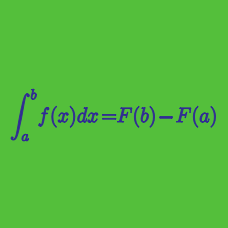Calculus

# Definite Integrals Warmup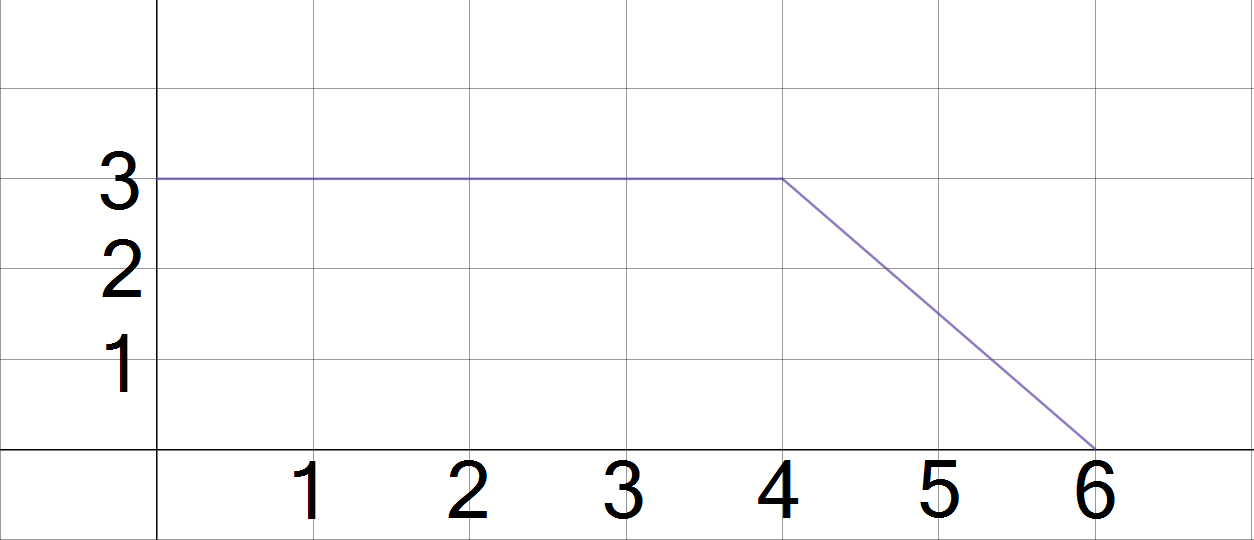The graph of the function $y = f(x)$ is shown above. What is the value of $\int_0^6 f(x) dx?$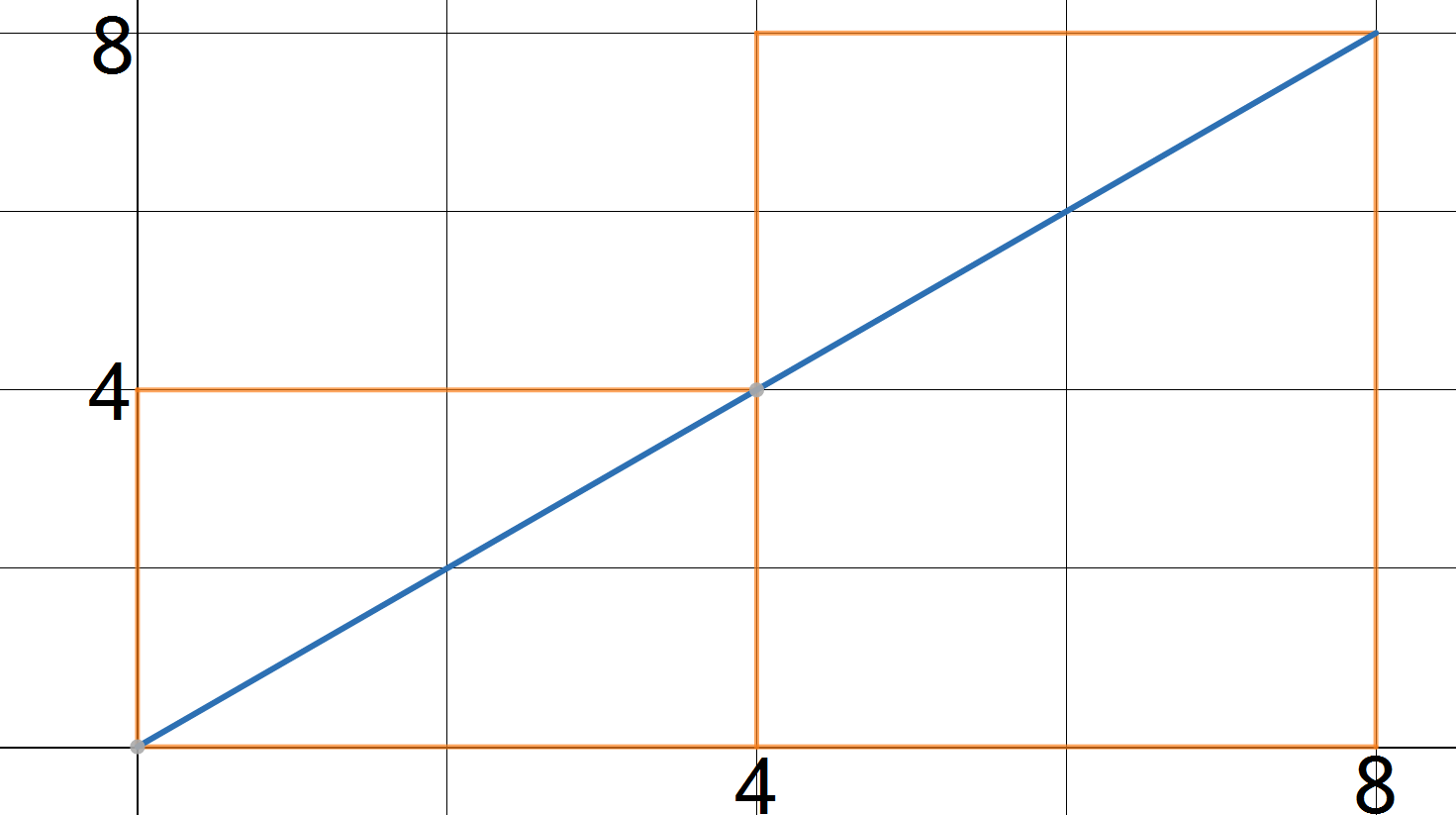Steve knows that if he uses right-hand endpoints with 2 subintervals to find a Riemann sum approximation of $\int_0^8 x dx,$ his approximation will be the sum of the areas of the 2 rectangles shown. Assuming all his work is correct, what will his approximation be?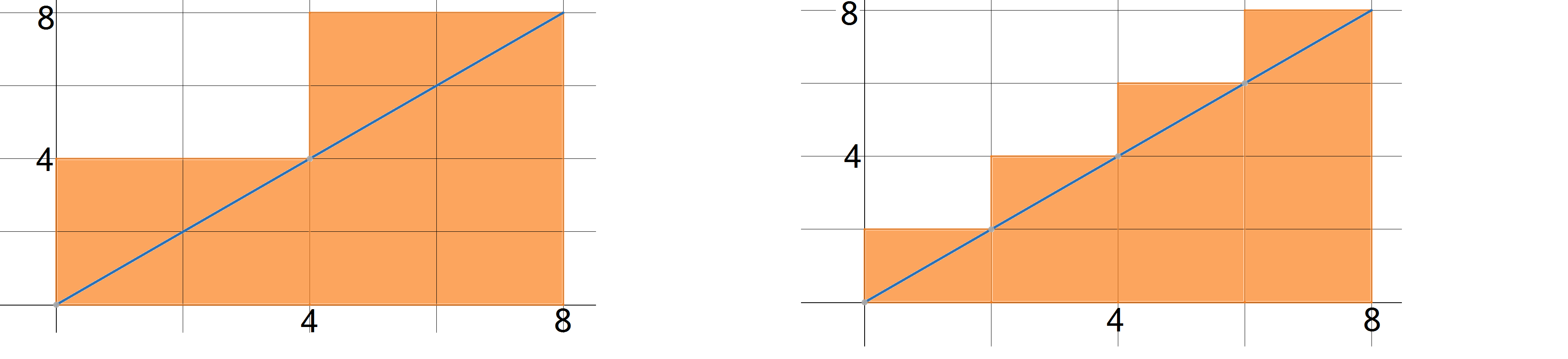Morgan and Pat use right-hand endpoints to find Riemann sum approximations of $\int_0^8 x dx.$

If Morgan uses 2 subintervals, and Pat uses 4 subintervals, whose approximation is closer to the true value of the integral?

Ferb knows that $\int_0^8 x dx = 32,$ and is interested in how close a right-hand Reimann sum approximation to the integral can be.

Based on his work so far, if Ferb uses 32 subintervals, what will his approximation be?

 Number of subintervals Approximation Error 1 64 32 2 48 16 4 40 8 8 36 4 16 34 2 32 ??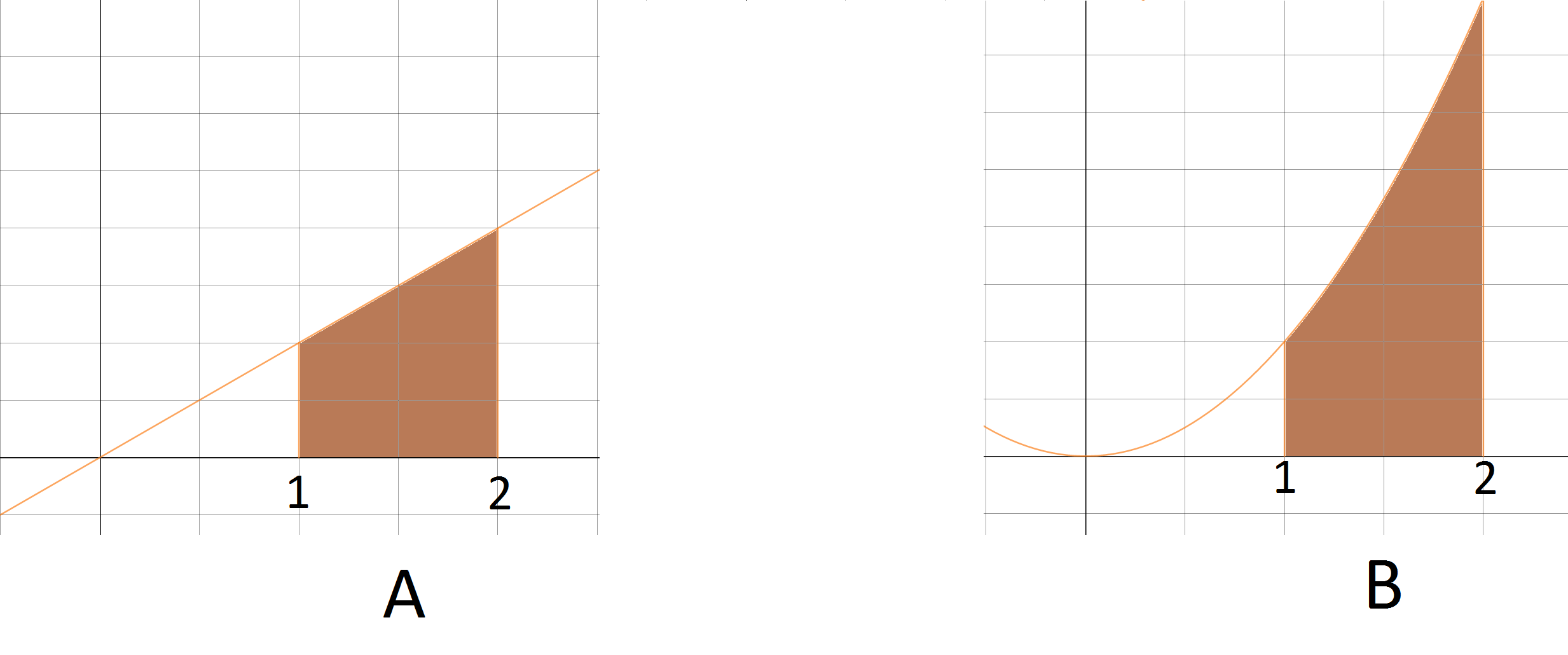$A = \int_{1}^{2} x dx, \,\,\,\, B = \int_{1}^{2} x^2 dx$

Which of the following is true of $A$ and $B?$

×